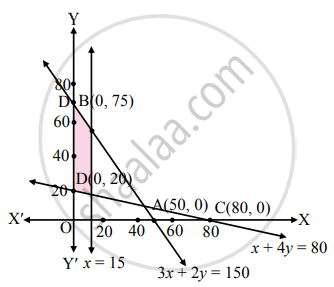Advertisement Remove all ads

# Solve the following system of inequalities graphically 3x + 2y ≤ 150, x + 4y ≥ 80, x ≤ 15, y ≥ 0, x ≥ 0 - Mathematics and Statistics

Graph

Solve the following system of inequalities graphically.
3x + 2y ≤ 150, x + 4y ≥ 80, x ≤ 15, y ≥ 0, x ≥ 0

Advertisement Remove all ads

#### Solution

To find graphical solution, construct the table as follows:

 Inequation Equation Doule Intercept form Points (x, y) Region 3x + 2y ≤ 150 3x + 2y = 150 "x"/50 + "y"/75 = 1 A (50, 0),B (0, 75) 3(0) + 2(0) ≤  150∴ 0 ≤  150∴ origin side x + 4y ≥ 80 x + 4y = 80 "x"/80 + "y"/20 = 1 C (80, 0),D (0, 20) 0 + 4(0) ≱ 80∴ 0 ≱ 80∴ non-origin side x ≤ 15 x = 15 – – 0 ≤ 15∴ L.H.S. of the linex = 15 x ≥ 0 x = 0 – – R.H.S. of Y-axis y ≥ 0 y = 0 – – Above X-axisThe shaded portion represents the graphical solution.

Is there an error in this question or solution?
Advertisement Remove all ads

#### APPEARS IN

Balbharati Mathematics and Statistics 2 (Commerce) 11th Standard HSC Maharashtra State Board
Chapter 8 Linear Inequations
Miscellaneous Exercise 8 | Q 14 | Page 122
Advertisement Remove all ads
Advertisement Remove all ads
Share
Notifications

View all notifications

Forgot password?